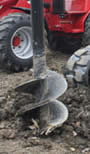# Builder's Mathematics

Here are some tips and tricks that may be useful when building.

## Need a Right Angle (90°) Fast ... ?

 Make a 3,4,5 Triangle ! Connect three lines: 3 long 4 long 5 long And we get a right angle (90°)### Other Lengths

You can use other lengths by multiplying each side by 2, or by 10, or any multiple:## Squaring and DiagonalHow do we ensure two sides are at right angles?

Run a diagonal.

But how long is the diagonal?The steps are:

• measure side a and square it (multiply it by itself)
• measure side b and square it also
• finish with square root

### Example: A frame with sides of 2.4 and 5.365

• 2.4 squared is 2.4×2.4 = 5.76
• 5.365 squared is 5.365×5.365 = 28.783225
• 5.76 + 28.783225 = 34.543225
• square root of 34.543225 is 5.877 (rounded to 3 decimal places)

And we get this:Perfection!

### Example: Sides are 300 and 450.5

• 300 squared is 300×300 = 90000
• 450.5 squared is 450.5×450.5 = 202950.25
• 90000 + 202950.25 = 292950.25
• square root of 292950.25 is 541.25 (rounded to 2 decimal places)
So the diagonal is 541.25

Notice how the squares can get very big, but come back to normal when we do the square root at the end

Try a few values here:

geometry/images/pythagoras-diag.js

Note: it is easy to slip a digit when doing these calcs, so double check!

Why does it work? It is Pythagoras' Theorem :In a right-angled triangle, the square of a (a2) plus the square of b (b2) is equal to the square of c (c2):

a2 + b2 = c2Take the square root of c2 to get c

So we square a, square b, add those to get c2:

## Filling Round Holes

A circle has about 80% of the area of a similar-width square:see circle area for exact values

So a circular hole has about 80% of the volume of a squared-off hole!### Example: You want to drill foundation holes and fill them with concrete.The holes are 0.4 m wide and 1 m deep, how much concrete should you order for each hole?They are circular (in cross section) because they are drilled out using an auger.

You can make an estimate by:

• 1. Calculating a square hole: 0.4 × 0.4 = 0.16 m2
• 2. Taking 80% of that (estimates a circle): 80% × 0.16 m2 = 0.128 m2
• 3. And the volume of a 1 m deep hole is: 0.128 m3

So you should order 0.128 cubic meters of concrete to fill each hole.

Note: a more accurate calculation using the circle's true area gives 0.126 m3

## Estimating Piles

A cone (such as a heaped pile of sand) has exactly one third of the volume of a surrounding cylinderA cone has about one quarter of the volume (closer to 26%) of a surrounding box with a square base:But be careful: if the base of the heap is much wider in one direction, then this estimate won't work well.